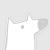ICS：International Conference on Supercomputing。 Explanation：国际超级计算会议。 Publisher：ACM。 SIT： http://dblp.uni-trier.de/db/conf/ics/

### 热门内容0250

### 最新内容000

### 最新论文In this paper we provide sub-linear algorithms for several fundamental problems in the setting in which the input graph excludes a fixed minor, i.e., is a minor-free graph. In particular, we provide the following algorithms for minor-free unbounded degree graphs. (1) A tester for Hamiltonicity with two-sided error with $poly(1/\epsilon)$-query complexity, where $\epsilon$ is the proximity parameter. (2) A local algorithm, as defined by Rubinfeld et al. (ICS 2011), for constructing a spanning subgraph with almost minimum weight, specifically, at most a factor $(1+\epsilon)$ of the optimum, with $poly(1/\epsilon)$-query complexity. Both our algorithms use partition oracles, a tool introduced by Hassidim et al. (FOCS 2009), which are oracles that provide access to a partition of the graph such that the number of cut-edges is small and each part of the partition is small. The polynomial dependence in $1/\epsilon$ of our algorithms is achieved by combining the recent $poly(d/\epsilon)$-query partition oracle of Kumar-Seshadhri-Stolman (ECCC 2021) for minor-free graphs with degree bounded by $d$. For bounded degree minor-free graphs we introduce the notion of covering partition oracles which is a relaxed version of partition oracles and design a $poly(d/\epsilon)$-time covering partition oracle. Using our covering partition oracle we provide the same results as above (except that the tester for Hamiltonicity has one-sided error) for minor-free bounded degree graphs, as well as showing that any property which is monotone and additive (e.g. bipartiteness) can be tested in minor-free graphs by making $poly(d/\epsilon)$-queries. The benefit of using the covering partition oracle rather than the partition oracle in our algorithms is its simplicity and an improved polynomial dependence in $1/\epsilon$ in the obtained query complexity.000下载预览
Top